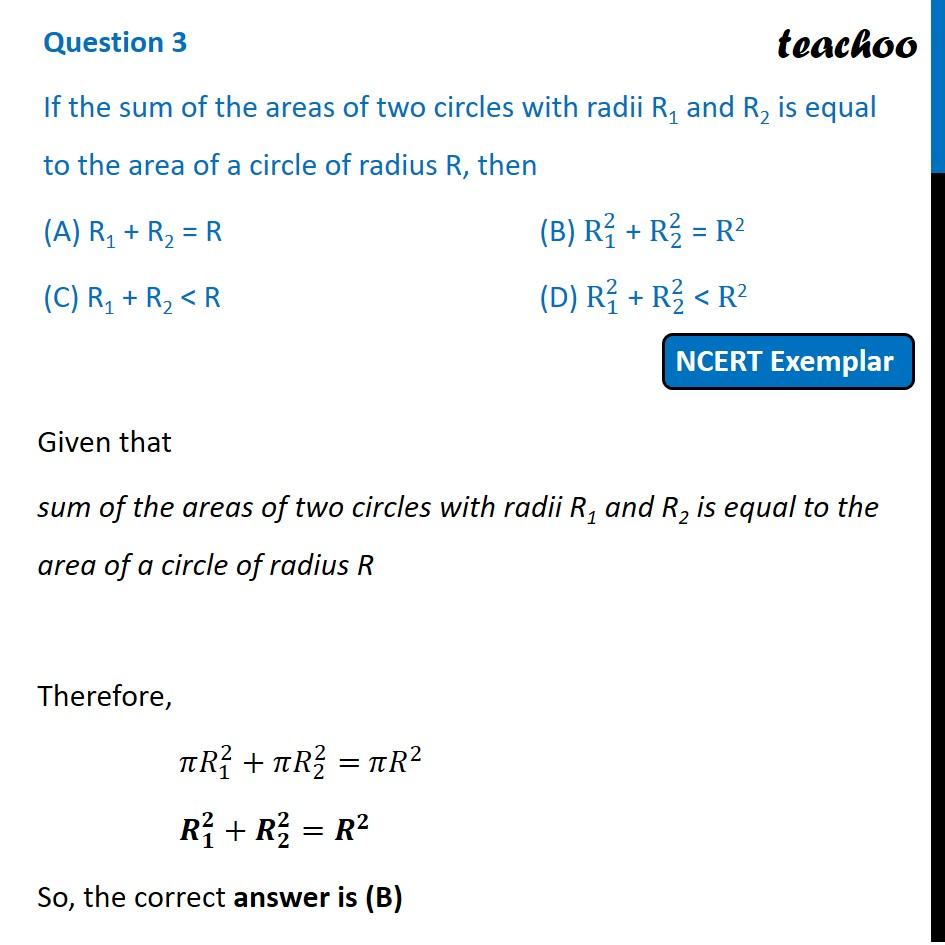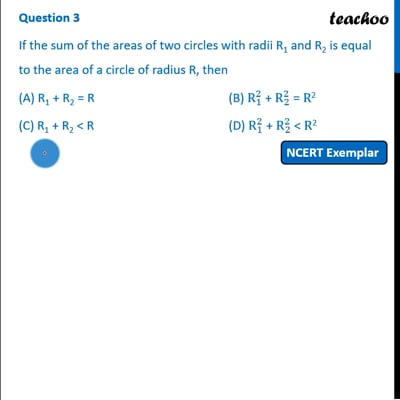NCERT Exemplar - MCQ

Chapter 12 Class 10 Areas related to Circles
Serial order wise

## (D) R 1 2 + R 2 2 < R 2This video is only available for Teachoo black users

Introducing your new favourite teacher - Teachoo Black, at only ₹83 per month

### Transcript

Question 3 If the sum of the areas of two circles with radii R1 and R2 is equal to the area of a circle of radius R, then (A) R1 + R2 = R (B) R_1^2 + R_2^2 = R2 (C) R1 + R2 < R (D) R_1^2 + R_2^2 < R2 Given that sum of the areas of two circles with radii R1 and R2 is equal to the area of a circle of radius R Therefore, 𝜋𝑅_1^2+𝜋𝑅_2^2= 𝜋𝑅^2 𝑹_𝟏^𝟐+𝑹_𝟐^𝟐=𝑹^𝟐 So, the correct answer is (B)